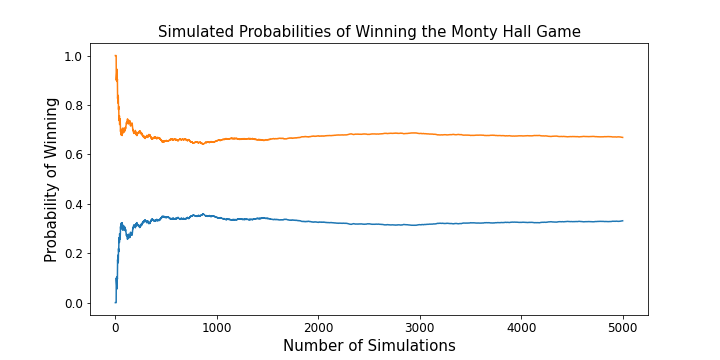They key to understand the 3 Doors or  Monty Hall game is to realize that we are choosing the wrong door with a much higher probability than the right one (66 vs 33% aprox), so if we are offered a second chance, the optimal strategy is to switch our initial choice.

The “Statistical Illusion”:
https://statisticsbyjim.com/fun/monty-hall-problem/

## Frequentist Simulations & Bayesian CalculationsWin probabilites for the two strategies of the Monty Hall game. Read on to find the optimal strategy.

## Introduction

Recently, I was asked to code a simulation of the Monty Hall game during a lesson on Bayesian statistics. I found it amusing that I was required to use a frequentist approach to probability for insight into what was explained as a Bayesian problem. I decided to Investigate further and come up with my own Bayesian understanding of what was going on.

For those unfamiliar with this famous mental puzzle, allow me to lay out the rules and key assumptions of the game. The Monty Hall problem is named after the host of the Let’s Make A Deal game show and follows similar rules to that game. A contestant is presented with identical three doors, two of which contain goats, and one of which contains a car. The contestant is then asked to announce which door they chose, and one of the other doors is opened. Its contents are revealed to the player, and the host then asks the player if they would like to switch their choice of doors before the car is revealed. Whichever choice the player makes at this point, they win if the car is behind the door they chose. You should take a moment to think about what you would do in this situation, and take note of it. You may be surprised, or have some of your assumptions challenged, by the end of the article.

## Random Choices

According to wikipedia, although the Monty Hall problem was originally proposed in 1975, it gained notoriety in 1990 after being posed as a question to a columnist in Parade magazine. The columnist’s correct solution sparked a harsh (and largely incorrect) backlash. Before diving into the simulation code and mathematical solution, let’s lay out some key assumptions of the game:

• The car is placed at random.
• The player chooses their door at random.
• The host always opens a door (which contains a goat) that the player didn’t choose. Also, The host makes this choice at random if the player chose the door containing the car at the beginning of the game.
• The player is always given the choice to change doors after the door containing the goat is opened.

These assumptions of random choice are used liberally in the simulation code, and also undergird the mathematical calculation later in the article. If these assumptions are changed, either the calculation would take on a different form, or the underlying probabilities would change. The Monty Hall problem

Source: towardsdatascience.com